# Data Conversion

## Data Conversion

In java programming we have six data conversion technique they are:.

### 1 Converting numeric string type data into numerical / fundamental type values

In order to convert numerical string into numerical or fundamental values we use the following generalized predefined method which is present in wrapper classes.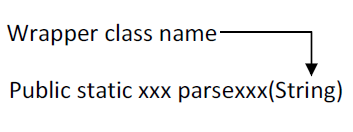Here xxx represent any fundamental data type.

## Example

```
String  s1="100";
int  x=Integer.parseInt(s1);
```

## Example

```
String  s2="100.75f";
Float  y=Float.parseFloat(s2);
```

## 2 Converting numeric / fundamental type values into string type values

In order to convert numeric / fundamental type values into string values, we use the following predefined static overloaded method.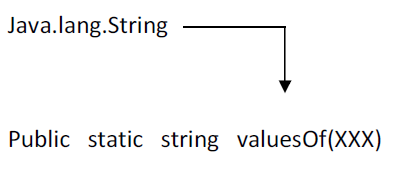Here XXX represent any fundamental data type values

## Example

```int a=10;
```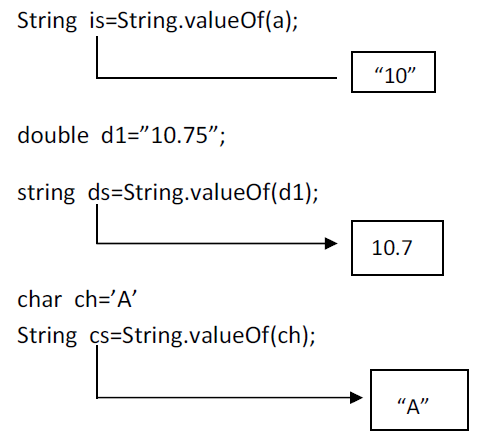## 3 Converting fundamental type values into object type values:

In order to convert the fundamental data into equivalent wrapper class object type data we use the following generalized predefined parameterized constructor by taking fundamental data type as a parameter.

Example: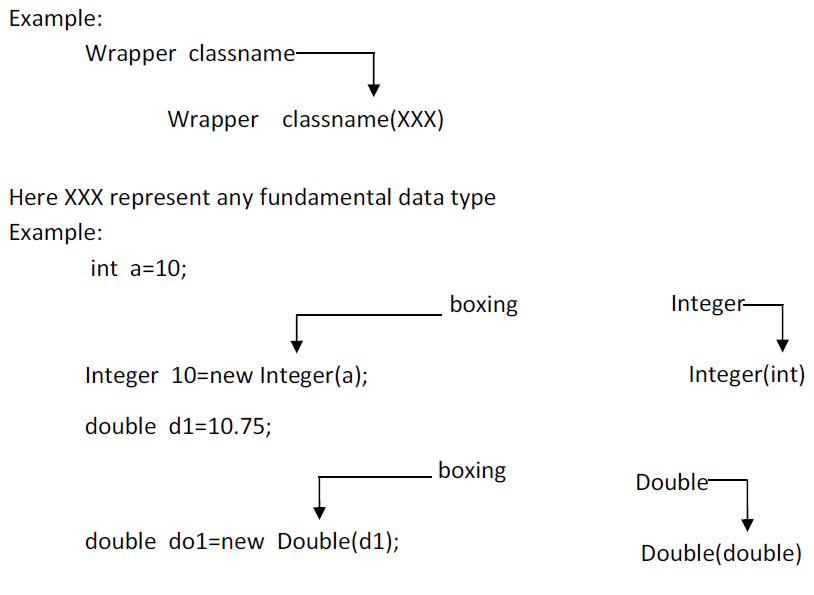in JDK 1.4 converting fundamental data type values into wrapper class object is known as boxing. In the case of JDK 1.5 and in higher version it is optional to the java programmer to convert fundamental data type value into equivalent wrapper class object. That is implicitly taken care by JVM and it is known as auto boxing.

## Definition of auto boxing

The process of implicitly converting fundamental type value into equivalent wrapper class object is known as auto boxing.

## 4 Converting object type value into fundamental type value:

In order to convert wrapper class object data into fundamental type data, we use the following predefined instance method present in each and every wrapper class.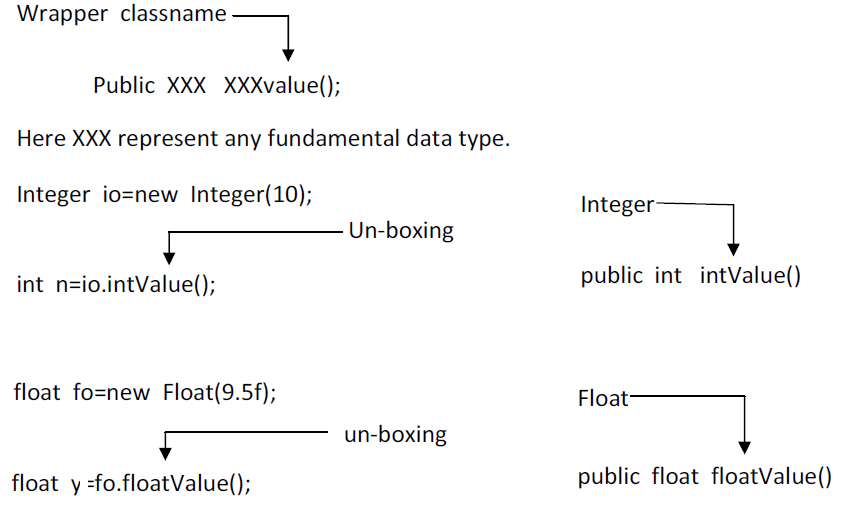In case of JDK 1.5 and in higher version it is optional to the java programmer to convert object data into fundamental type data and this process is known as auto un-boxing and its takes care by JVM.

## Definition of auto un-boxing

In process of implicitly conversion objects type data into fundamental type data is known as auto un-boxing.

## 5 Converting String type data into object type data

In order to convert String type numeric data into equivalent wrapper class object, we use the following predefined parameterized constructor by each and every wrapper class except character class.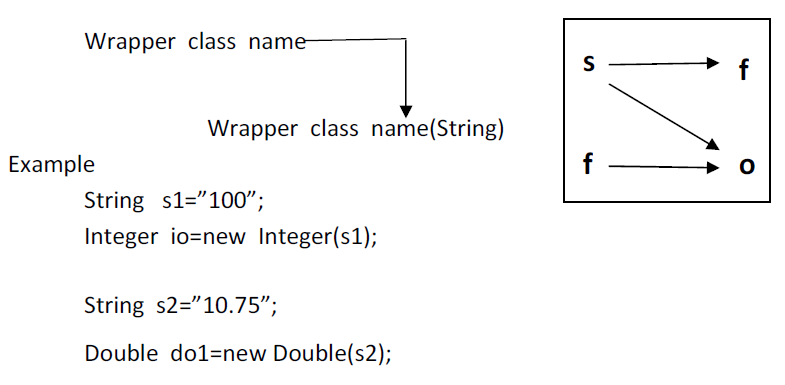## 6 Converting wrapper class object type data into String type data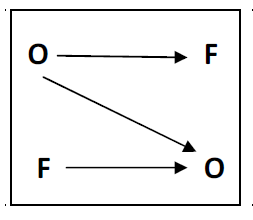To convert wrapper class object type data into string type data we use the following generalized predefined instant method which is present each and every wrapper class.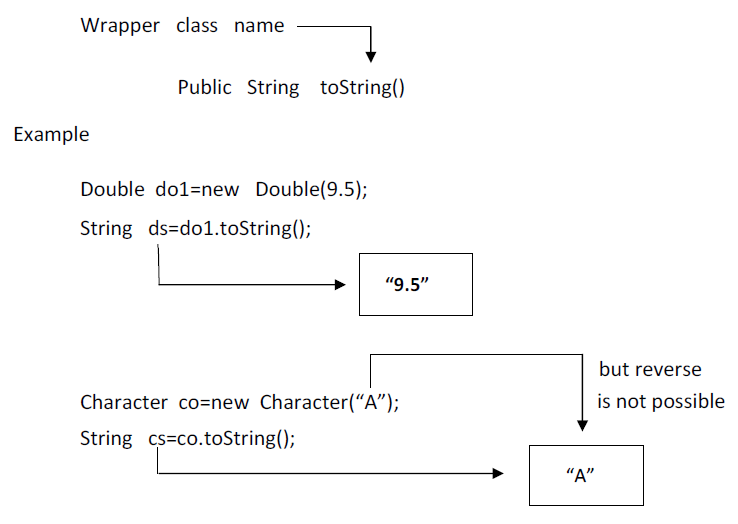Example

int a=10;

• String is=String.valueOf(a);
• Integer io=new Integer(is);
• int x=io.intValue();
• Integer io=new Integer(n);
• String so=io.toString();
• int x=Integer.parseInt(so);